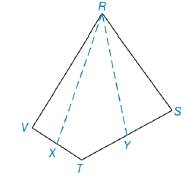Chapter 8.1, Problem 32EElementary Geometry For College St...

7th Edition
Alexander + 2 others
ISBN: 9781337614085

Solutions

Chapter
SectionElementary Geometry For College St...

7th Edition
Alexander + 2 others
ISBN: 9781337614085
Textbook Problem

For Exercise 31 and 32, X is the midpoint of V T ¯ and Y is the midpoint of T S ¯ . See the theorem in Exercise 30.If A R Y T X = 13.5  in 2 , find A R S T V .Exercises 31, 32

To determine

To find:

ARSTV If ARYTX=13.5 in2.

Explanation

“A median of a triangle separated it into two triangles of equal area”.

Calculation:

Given,

Area of RYTX(A)=13.5 in2

Draw a diagonal RT¯ in RSTV which separates RSTV into two triangle RVT and RVT

In RVT X is the midpoint of VT¯ from R

(i.e.) RX is the median of RVT since, “A median of a triangle separated it into two triangles of equal area”

(i.e.) RX¯ separates the RVT into RXV and RXT with equal area,

ARXV=ARXT

In RTS, Y is the midpoint of TS¯ from R.

(i.e.) RY¯ is the median of RTS

RY¯ separates the RTS into RYT and RYS with equal area,

ARYT=ARYS

Now, the figure becomes,

Still sussing out bartleby?

Check out a sample textbook solution.

See a sample solution

The Solution to Your Study Problems

Bartleby provides explanations to thousands of textbook problems written by our experts, many with advanced degrees!

Get Started

Convert the expressions in Exercises 6584 to power form. 3x1.2213x2.1

Finite Mathematics and Applied Calculus (MindTap Course List)

In Problems 1-6, graph each inequality. 5.

Mathematical Applications for the Management, Life, and Social Sciences

sinx=ex+ex2

Study Guide for Stewart's Single Variable Calculus: Early Transcendentals, 8th

For

Study Guide for Stewart's Multivariable Calculus, 8th